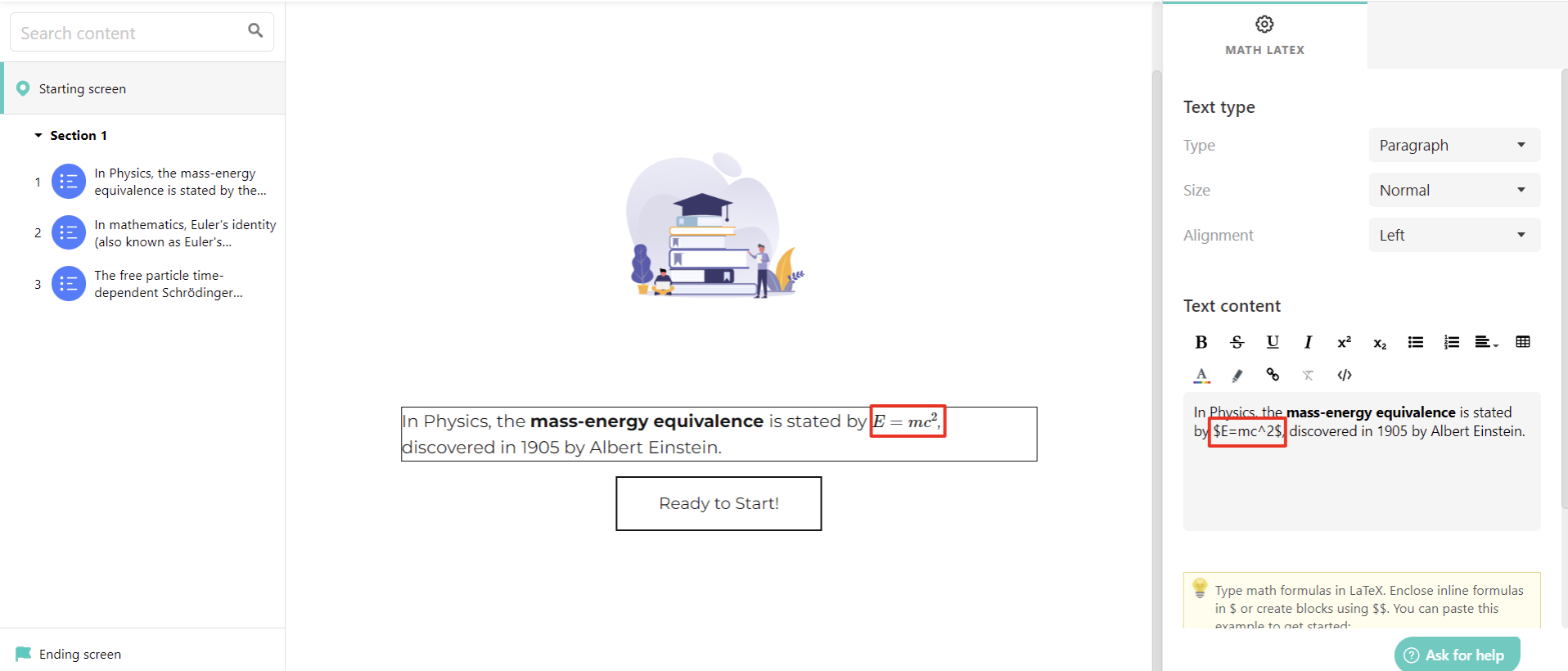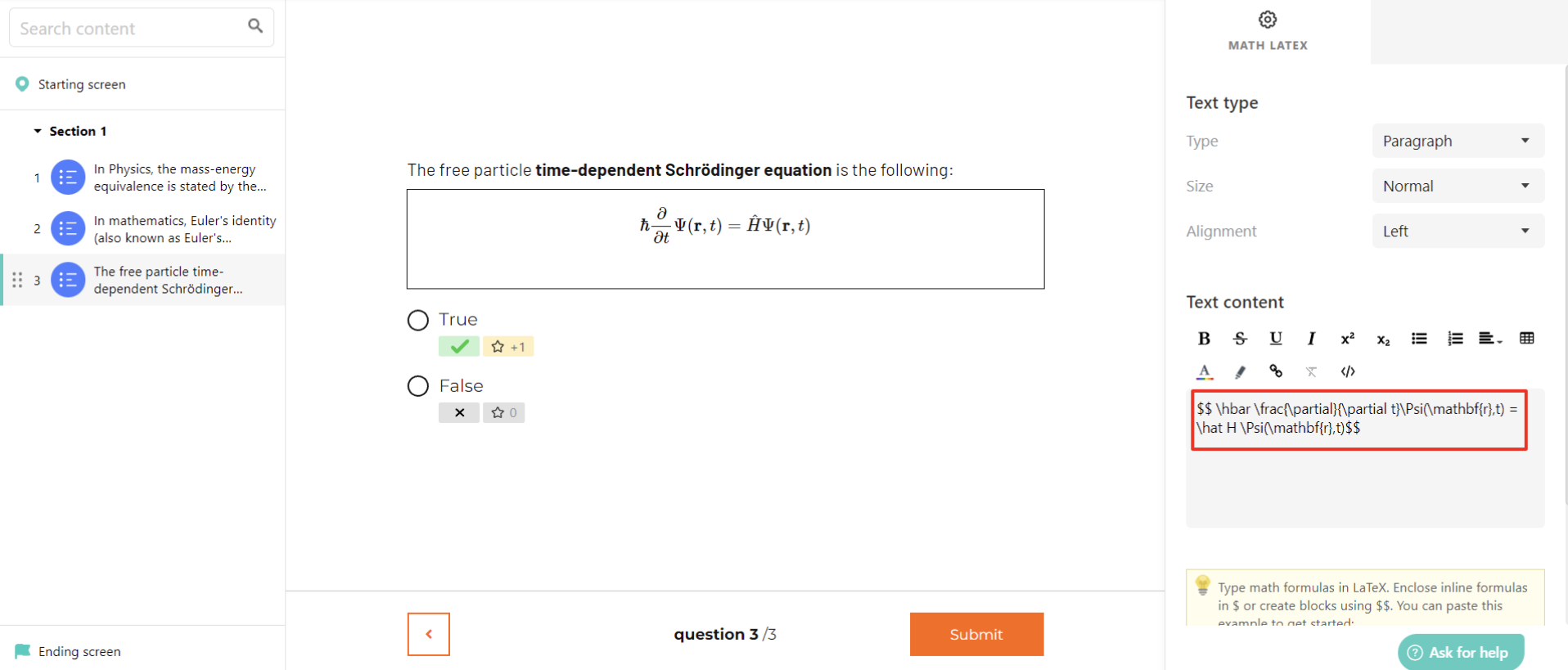In our Assessment builder, it is possible to create assessments containing mathematical expressions - equations, with the use of the Math Latex text widget, that can be added:

• On the Starting or Ending page.
• In Question descriptions.
• As an option in the Custom Multiple Choice/Multiple Choice-Multiple Answers types of questions.

To add a Math Latex text widget:

1. Click on the plus icon (in the starting/ending screen, in the description of a question, or in the Custom MC/MCMA types of questions).

2. Add the relevant Math LaTeX x + y = 0 widget under Main Text.In LearnWorlds, we support the rendering of mathematical expressions formatted in LaTeX, as supported by MathJax.

It is possible to add the mathematical expression or equation both inline wrapped between a single $(for example,$E=mc^2$).You can block mathematics wrapped between $$(for example$$ \hbar \frac{\partial}{\partial t}\Psi(\mathbf{r},t) = \hat H \Psi(\mathbf{r},t)$$).Only the mathematical expression should be wrapped between the$ sign, as spaces entered between them are ignored (per Latex's formatting).The math widget’s contents are rendered in all forms of web Feedback however, in the PDF feedback, it displays as the following: [math]. This is also the case for the export of the answers and the Responses page.

Did you find it helpful? Yes No

Send feedback